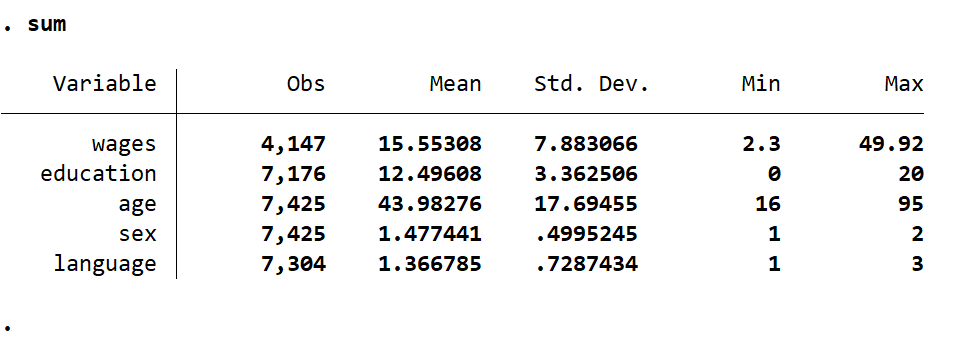Skip to main content
It looks like you're using Internet Explorer 11 or older. This website works best with modern browsers such as the latest versions of Chrome, Firefox, Safari, and Edge. If you continue with this browser, you may see unexpected results.

# Stata

A brief introduction to Stata

## Descriptive Statistics for One Variable

Getting the descriptive statistics in Stata is quick for one or multiple variables. Descriptive statistics are measures we can use to learn more about the distribution of observations in variables for analysis, transforming variables, and reporting. Each descriptive statistic has their own formula that we will not be covering in this guide, but we will walk through the interpretation of each.

Below is the code for calculating the descriptive statistics of the variable wages.

Code

sum wages

We are calculating the sum (short of summary) of the variable wages

OutputA

The output chart shows us the name of the variable we are examining and the descriptive statistics. There is one row in the chart, wages, which is of observations without missing data in this dataset.

Moving from left to right, the variable name is found under the Variable column.

Next, there are 4,147 observations without missing data for the variable wages.

The average wage value in this dataset is 15.5531 which is below the middle value of 26.11 ((49.92 – 2.30)/2) (not shown), indicating the distribution of the data is skewed toward lower values.

The standard deviation is 7.887, indicating there is a wide distribution of the data.

The minimum value recorded among the observations is 2.30 and the maximum is 49.92. Quite the range!

## Descriptive Statistics for Multiple Variables

We can also calculate the descriptive statistics for all the variables in one command line.

Code

sum

With this code we are calculating the descriptive statistics for the variables wageseducationagesex, and language

Output